By: Andrés Felipe Echeverri Guevara

Since the Kalman Filter came out in the ’60s, it has been extensively studied and researched due to the advances in digital computing and algorithms. Several have been the techniques proposed in the literature that aims to improve it and, in this post, we will explore the issues of outliers; while some more recent filters such as the Particle Filter are further robust to outliers, which implement higher demands of computational capabilities. Kalman Filter can have similar results as the Particle filter with right tuning, model selection and outliers detection/rejection mechanism. Anomalies on measurements can be solved by using an approach such as the Mahalanobis distance. Moreover, we will focus on its implementation rather than deriving the whole Kalman Filter. A general overview is given with an example that uses a generic signal with a one-dimensional transition matrix.

The following graph shows a damped signal, which is a common signal in many applications.Before presenting the Kalman filter equations, it is necessary to first define a state-space model of the system. In this implementation, let’s assume that our state vector x = [s]. Where s is a signal acquired from a sensor. This sensor could be an ultrasound measuring distance from a quadcopter, or the voltage in a noisy RC circuit, whatever application you have in mind, you name it, for our general purposes, let’s use a signal with some noise and outliers added.

Let’s analyze a portion of the signal, in order to find some statistics about it.The standard deviation is around 0.10-0.15, a value that can be used after. However, this is just an insight. Tunning the filter will be accomplished by how much lag we are able to accept in the implementation, or how fast we want the filter to respond. Let’s now start defining our Kalman Filter.

The following equations represent the dynamics and the measurement model respectively.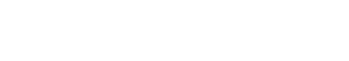These equations include the state transition matrix A, the influence of the control action B (not implemented in our case), the process noise w, the observation matrix C and the measurement noise v. The process noise is white, Gaussian, with variance Rww and measurement noise, is white, Gaussian with variance Rvv. Some other conventions can be found in the literature. The standard Kalman filter is comprised of two major components and three intermediary calculations. Let’s initialize these values.

The two major components are a prediction step and an update step. The update step refines or corrects the previous prediction. The three intermediary calculations (innovation, error covariance, and Kalman gain), are necessary for moving from the prediction step to the update step. Below are all the necessary equations for implementing the standard Kalman filter:

## Prediction## Innovation## UpdateThe Kalman filter, like any other filter, is susceptible to outliers. An outlier can be understood as a behavior that is not considered in the model. Several mechanisms have been proposed and one of them easy to implement is the Mahalanobis Distance (MD). The MD can be easily explained using point P with coordinates (x,y) and joint distribution of two variables defined by parameters μ, σx and σy. The distance is zero if P=μ. The distance increases as P moves away from μ. Evidently, this method can also be used for more than two dimensions.To calculate the MD, one can use the predicted measurement to determine outliers. This error is then defined as follow: given a measurement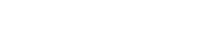the MD from this measurement to a group of predicted values with mean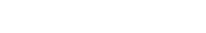and innovation covariance matrix Ree is given by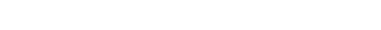The MD can be approximated by the following equation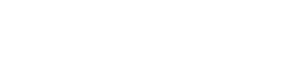Where: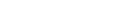And Reei is the ith element along Ree

## Weighted Mahalanobis Distance

How could we use the Mahalanobis Distance in order to remove the outliers? Some literature uses it by applying a hard limiter; if the values lie outside the ellipse, do not perform any update and keep the previous values. However, I have come up with a different approach that allows us to keep doing updates, a more elaborated description and implementation can be found in the following paper. Let’s sum it up briefly.

Before we talk about how the MD is used to remove outliers, let’s talk briefly about the Rww and Rvv values. Finding the right values is often difficult in most applications. We could rely on the statistics of the system, but a dynamic system makes it difficult to accomplish such a task. How about if we see these as tunning parameters? Rvv is the measurement noise covariance, it can be considered as how much the system can trust on the measurement readings, the bigger the Rvv, the lesser the trust the system will have on the sensor measurement. Similar behavior can be seen by using Rww, which is the process noise covariance and is related to the amount of noise on the system’s model. It can be conceived how much I trust the model, let’s assume that I have a car, if the speed is a measurement used in the model, there will be variations due to bumps, hills, winds and some other factors, these are the different causes that could affect the model. The bigger the Rww value is, the lesser the confidence in the model.

The MD comes handy on the Innovation calculation. If there is an anomaly that was not considered on the model, the MD will penalize such anomalies for not being present on the model by using a sigmoid function.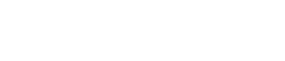Where ξ is a constant and the MD starts being penalized. Once the MD is found, a new Rvv can be calculated, one that is proportional to the amount of noise suffered by the sensor.As it is noticeable from the previous graph, the MDw will be able to detect the anomalies and when they happen.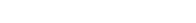Where Δ is a constant that penalizes the Rvv. In some other words, we can adapt Rvv based on sensor performance, giving us the ability to adaptively trust more or less in the sensor model.Let’s see the difference between the original signal and the filtered one.## Summing up

While the regular Kalman filter works well, it fails sometimes with anomalies that are not considered in the model. Better filters could be implemented but at a higher computational cost such as the Particle filter, which is better at handling outliers. The Extended Kalman filters are also good, but they will also fail at some point.

You might be asking yourself. Where I could apply it? Filtering is widely applied in numerous fields. Guidance, navigation, control, econometrics and myriad more. I have used it in several applications myself, from BLE application to filter out thE RSSI up to Drone odometry and guidance, IMU sensor fusion and some others. Whatever application that produces a time series, Kalman Filter comes in handy to remove noise and detect outliers.

Moreover, the previous implementation can be further extended to any application, the state vector can be designed with the dimension that meets your needs.

You can find the code in the following link.

Kalman Filter used for sensor fusion and target following